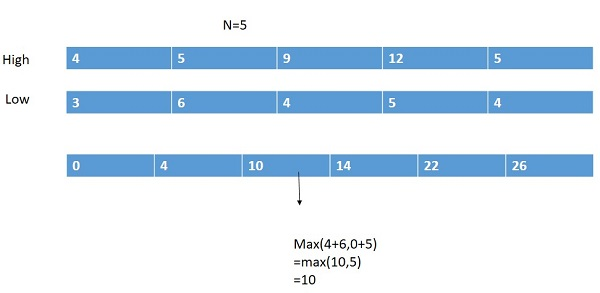# High-effort vs. Low-effort Task Problem

Here, we are going to learn the solution of high-effort vs. low-effort task problem using dynamic programming.
Submitted by Souvik Saha, on June 25, 2020

Problem statement:

A list of n days is given to you and there are three columns. One is the day, the second one is high-effort value, and the another one is low-effort value. At a time you can pick only one either high-effort value or low-effort value. You can pick a high-effort task only when you don't take any of the tasks at the day before. You have to find out the maximum task is possible in n days.

```Input:
T Test case
T no. of input string will be given to you.

E.g.
2

Day	High	Low
1	4	3
2	5	6
3	9	4
4	12	5
5	5	4

Day	High	Low
1	2	3
2	4	2
3	7	8
4	9	7
5	10	6

Constrain:
1≤ n ≤50

Output:
Print the maximum task is possible in n days.
```

Example

```T=2

Input:
5
4 5 9 12 5
3 6 4 5 4

Output:
26 ( 4+ 6+ 12+ 4)

Input:
5
2 4 7 9 10
3 2 8 7 6

Output:
26 (3+2+8+7+6)
```

Explanation with example:

To solve this task problem using dynamic programming approach, we consider these two conditions,

1. If we will choose the low effort task then we have to add up this value with the value at (i-1)th index of the calculating array.
2. If we will choose the high effort task then we have to add up this value with the value at (i-2)th index of the calculating array.

After getting the value we will choose the maximum value between them.

Example:

```5
4 5 9 12 5
3 6 4 5 4```

C++ Implementation:

```#include <iostream>
using namespace std;

int max_amount(int* high, int* low, int n)
{
int arr[n + 1] = { 0 };
arr = max(high, low);

for (int i = 2; i <= n; i++) {
arr[i] = max(arr[i - 1] + low[i - 1], arr[i - 2] + high[i - 1]);
}

return arr[n];
}

int main()
{
int t;

cout << "Test Case : ";
cin >> t;

while (t--) {
int n;

cout << "Enter the number of elements : ";
cin >> n;

cout << "Enter the High values : ";
int high[n], low[n];
for (int i = 0; i < n; i++) {
cin >> high[i];
}

cout << "Enter the low values : ";
for (int i = 0; i < n; i++) {
cin >> low[i];
}

cout << "Maximum values : " << max_amount(high, low, n) << endl;
}

return 0;
}
```

Output

```Test Case : 2
Enter the number of elements : 5
Enter the High values : 4 5 9 12 5
Enter the low values : 3 6 4 5 4
Maximum values : 26
Enter the number of elements : 5
Enter the High values : 2 4 7 9 10
Enter the low values : 3 2 8 7 6
Maximum values : 26
```